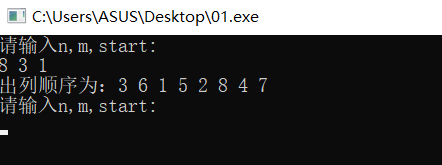2021-03-21 00:39

# 约瑟夫问题C++ （萌新学习c++）

``8 3 1``

````7`
```

``````
#include <iostream>

void JosePhus(int n, int m, int start) {
int i, *arr = new int[n]; // 动态分配数组
int count; // 保存当前已站出来的人数

for(i = 0;i < n; i++) // 初始化，把各位置号存入数组中
arr[i] = i + 1;
count = 1;
start--; // 因为数组下标从0开始，所以要减1
while(count < n) { // 当前已站出来的人数
std::cout << arr[start] << ","; // 输出当前要站出来的人的位置号
for(i = start; i < (n - count); i++)
arr[i] = arr[i+1]; // 把位置号前移

// start + m - 1:减1是因为该位置号的人已出列，位置号前移了一位
// n - count: 当前剩下的人数
start = (start + m - 1) % (n - count); // 考虑到尾了，要从头开始，所以用取余，或者用判断方法

count++;
}
std::cout << arr << "\n";
}

int main(int argc, const char * argv[]) {
int n, m, start; // n:人数  m:数到多少出列  start:开始位置

std::cout << "请输入n,m,start:\n";
while(std::cin >> n >> m >> start) {
JosePhus(n, m, start); // 调用解决约瑟夫问题的函数
std::cout << "请输入n,m,start:\n";
}
return 0;
}
``````
• 点赞
• 写回答
• 关注问题
• 收藏
• 邀请回答

#### 2条回答默认 最新

•小白小白你好菜 2021-03-21 09:29
已采纳
``````#include <iostream>

void JosePhus(int n, int m, int start) {
int i, *arr = new int[n]; // 动态分配数组
int count = 1;; // 保存当前已站出来的人数
for(i = 0;i < n; i++) // 初始化，把各位置号存入数组中
arr[i] = i + 1;
int sum = n;
//输出参考    printf("出列顺序为：");
while(count < n) { // 出列顺序
start--; // 因为数组下标从0开始，所以要减1
int index = (start+m-1) % sum;//记录第count次出列的下标
//输出参考  std::cout << arr[index] << " "; // 输出当前要站出来的人的位置号
for(i = index; i < sum-1; i++)//出列相当于人数少了一个，循环上限为sum-1
arr[i] = arr[i+1]; // 把位置号前移
start = index + 1;//第count+1次开始位置为上次出列下标位置+1
sum--;//总人数减一
count++;//出列人数+1
}
std::cout<< arr <<"\n";//最后剩下的第一个元素为最后留下的人
}

int main(int argc, const char * argv[]) {
int n, m, start; // n:人数  m:数到多少出列  start:开始位置

std::cout << "请输入n,m,start:\n";
while(std::cin >> n >> m >> start) {
JosePhus(n, m, start); // 调用解决约瑟夫问题的函数
std::cout << "请输入n,m,start:\n";
}
return 0;
}``````
点赞 1 评论
•小白小白你好菜 2021-03-21 09:30点赞 1 评论